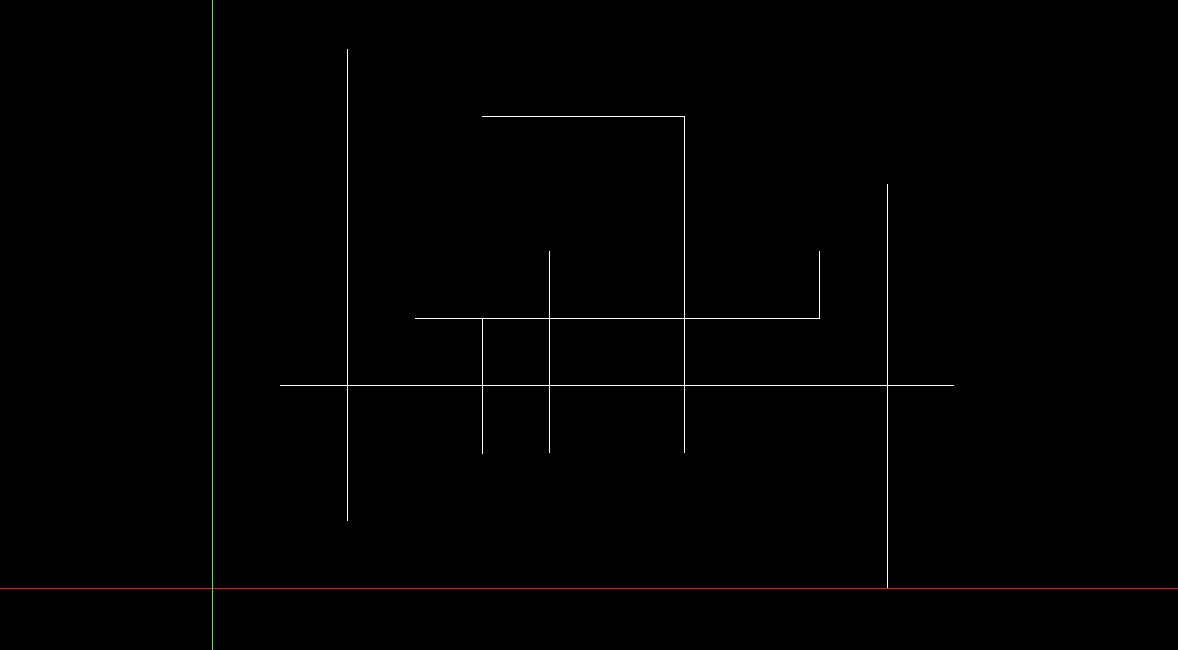# Segment intersections | Line sweep algorithm

geometry# # Usage

Given vertical or horizontal segments, report the number of intersections of them.

# # Algorithm

• Add following properties to each point.
• type: bottom or top of vertical segments (DOWN, UP). left or right of horizontal segments (LEFT, RIGHT).
• length: the length of segment which the point belongs to.
• Sort all the points according to the following:
• y ascending
• if y is a tie, type ascending(DOWN -> LEFT -> UP -> RIGHT)
• if type is a tie, x ascending
• Initliaze counter with 0
• Execute followings for each point
• type is UP) Insert x coordinate to Binary Tree BT
• type is LEFT) Add the number of elements to counter which is within x coordinate and x + length.
• type is DOWN) Erase x from BT

# # Time Complexity

$O(N \log N)$ for sorting points.

After that, insertion, deletion and search of set are $O(\log N)$.
Repeat it $N$ times. $N$ stands for the number of points.

$O(N \log N)$

# # Code

enum PointType {
DOWN, LEFT, UP, RIGHT // The order matters.
};

class Point {
public:
Vector2 coord;
PointType type;
Int length;

bool operator < (const Point &p) {
// if y is a tie, use type.
return (p.coord.y == coord.y && type != p.type)
? type < p.type
: coord < p.coord;
}

bool operator == (const Point &p) {
return coord == p.coord && type == p.type;
}
};

Int line_sweep() {
set<Int> BT;
Int count = 0;
sort(span_all(points));

for (auto p: points) {
switch (p.type) {
case DOWN:
BT.insert(p.coord.x);
break;
case LEFT:
count += distance(
lower_bound(span_all(BT), p.coord.x),
upper_bound(span_all(BT), p.coord.x + p.length)
);
break;
case UP:
BT.erase(p.coord.x);
break;
case RIGHT:
// do nothing
break;
}
}
return count;
}Remote freelancer. A web and mobile application enginner.
Traveling around the world based on East Asia.
I'm looking forward to your job offers from all over the world!

Offer jobs or contact me!